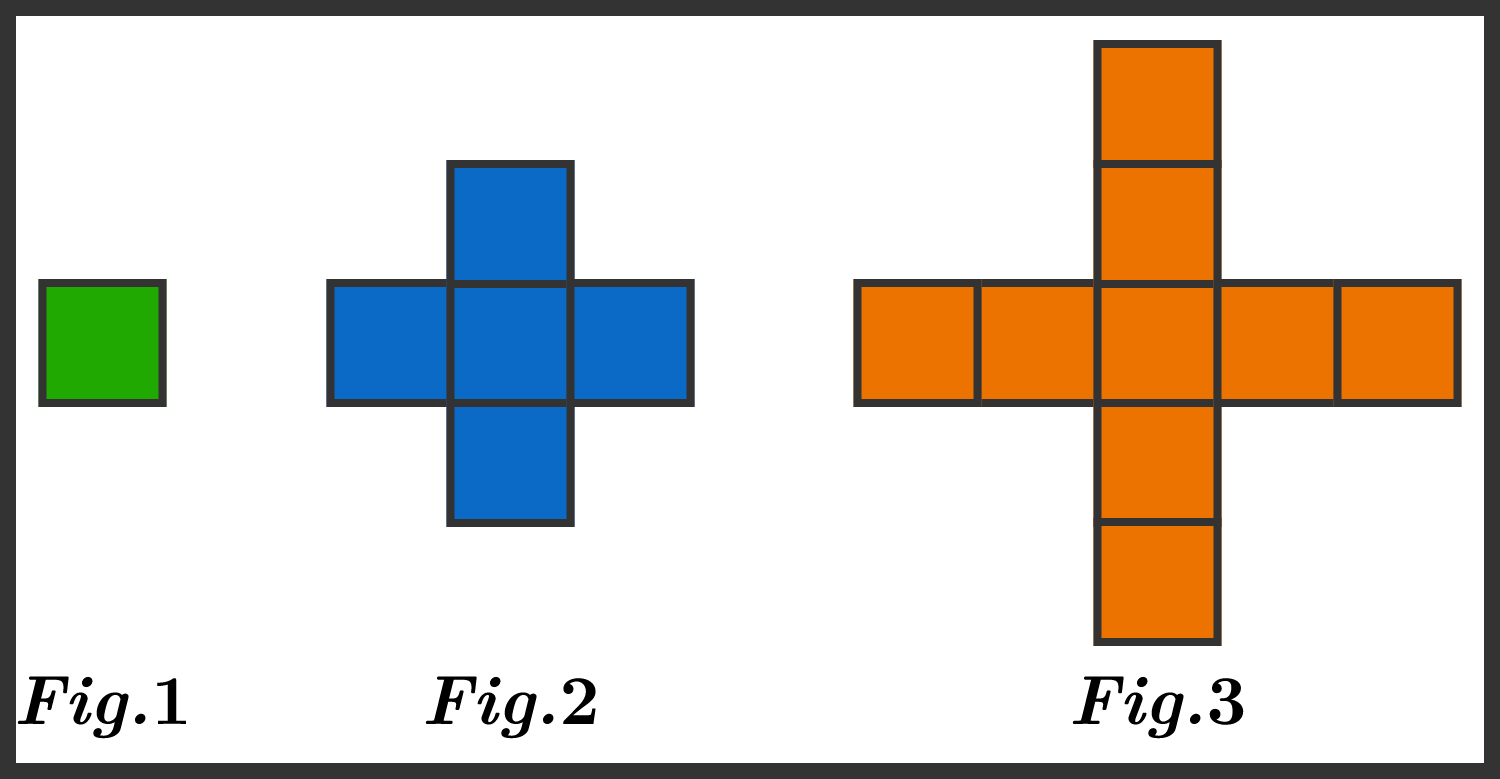# Perimeter Patterns

Geometry Level 1By tracing the edges, we can calculate that the perimeter of figure 1 is 4 units, and the perimeter of figure 2 is 12 units. What is the perimeter of figure 3?

×